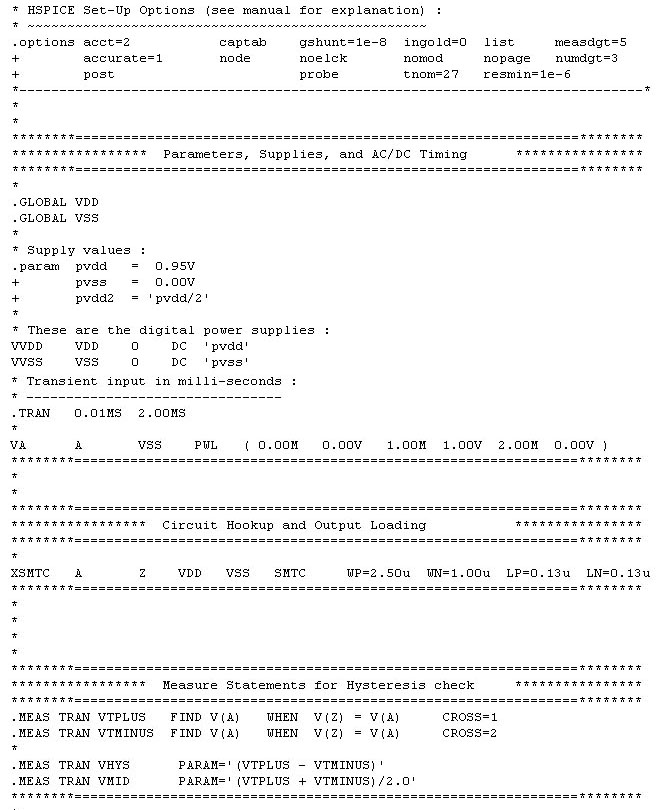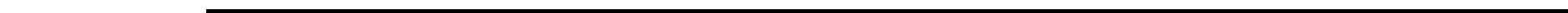Welcome to Spiceguy.net.
Today is: Tuesday March 21, 2023

Hysteresis Simulation

# This page illustrates how to Simulate a Schmitt Buffer using HSPICE and Plot the Hysteresis Loop using (slow) Transient or DC Analysis.

1. Rather than using DC analysis, we can also use Transient Analysis with a .tran in mS (milli-seconds).  This allows using transient measure statements to measure the "DC" trip points since time unit is milli-seconds.   (From experience, this transient method in mS gives same result as a straight-forward DC analysis)

2. Use the max voltage as 1/2 your tstop in mS.    e.g.: if you have a 1-volt buffer, run the analysis for 2mS with 0.01m tstep

Ex:     .tran 0.01mS   2.0mS

1. Use a PWL input to create a triangle input source with a peak of buffer's max voltage (1V) :

Ex:     VA    A    0       PWL ( 0.00m   0.00V    1.00m    1.00V     2.00m    0.00V)

1. Add measure statements to calculate input voltage when it crosses your output.
These two crossings are the positive & negative-going input levels (VT+ and VT-).
Ex:   (assumes A=Input,    Z= Inverting Output) :

.MEAS TRAN  VTPLUS       FIND V(A)     WHEN V(Z) = V(A)            CROSS=1
.MEAS TRAN  VTMINUS   FIND V(A)     WHEN V(Z) = V(A)            CROSS=2
.MEAS TRAN  VHYS            PARAM= '(VTPLUS - VTMINUS)'

The above measure statements will generate the hysteresis levels numerically.  To see these results graphically with the familiar hysteresis loop, see these images below for illustration:

A)  This image shows our transient simulation with TIME on the X-Axis.  We don't get the familiar hysteresis loop, but it's exactly what we  simulated with the triangle input.   You can graphically measure the trip points where the input crosses the output, or V(A) = V(Z).

(TRAN. ANALYSIS: Click image to enlarge)

B)  This image has instructions you need in AvanWaves to change your X-Axis from "Time" to Voltage V(A) using alternate DC analysis.   Repeat this process for all runs and plot V(A) on the X-Axis,  and then select V(Z) for each run.

(DC ANALYSIS: Click image to enlarge)

C)  Above procedure can be repeated to generate desired plot with hysteresis loops for all runs.   Below this plot follows a table of measured hysteresis levels (from HSPICE output) for my nine chosen process/voltage/temperature (PVT) conditions.

Note: Skewed process models were used for worst case trip variations with P-Channel stated first, Ex: SLOW-SLOW means slow PCH/slow NCH.

(DC HYSTERESIS PLOT: Click image to enlarge)

D)  Shown below is a portion of the HSPICE source deck used to generate these plots.  First one is transient, and the second is DC analysis.Transient analysis code (click to enlarge)                           DC analysis code (click to enlarge)

Note : Above simulation is also good for checking trip levels of gates without hysteresis.  Thus, you can use this setup to see if there is any hysteresis on a gate.  If not, then you get equal positive (VT+) and negative (VT-) trip points (i.e., with VHYS = 0).  For gates with more than one input, remember to repeat the trip measurement for each input individually since trip points will vary depending how far that input device is from the output.  See below for Nand gate structure.Nand Gate StructureSend email to Doran@Spiceguy.net with questions or comments about this web site.  Copyright © 2011 by Spiceguy.net.  Last modified: 12/09/11